# Image Generation Using Diffusion Models¶

In :
%pylab inline
from ipypublish import nb_setup

Populating the interactive namespace from numpy and matplotlib


## Introduction¶

One of the important applications of DLNs has been image generation. We already saw a few examples of this in Chapter ConvNetsPart2, where a ConvNet trained using Supervised Learning was run "backwards" in order to generate images. Given a set of training samples $\{X_1,...,X_N\}$, a more preferred approach to generating a new datapoint $X = (x_1,...,x_n)$ is to first estimate the distribution $p_\theta(X)$ that approximates the actual distribution $p(X)$ that the training samples come from (using Unsupervised Learning) and then sample from $p_\theta(X)$ to generate a new $X$. There are several ways in which this program can be put into practice:

• Autoregressive Generation: This technique works by building a model for the parametrized distribution $p_\theta(X)$ that maximizes the log likelihood of the observed data points $\{X_1,...,X_N\}$, and then generates new datapoint $X=(x_1,...,x_n)$ by making use of the recursion $$x_i = argmax_i\ p_\theta(x_i|x_1,...,x_{i-1})$$ This technique is more commonly used in NLP applications, and we saw several examples of this in Chapters NLP and Transformers, where the $p_\theta$ was implemented using a RNN/LSTM or Transformer. Images can also be generated by using this technique, using algorithms such as pixel-RNN or pixel-CNN. The main issue with using autoregressive image generation models is that they do generation on a pixel by pixel basis. Given a typical RGB image contains a few hundred thousand pixels, this can be a slow process.

• Generative Adversarial Networks (GANs): A much improved way of generating images was discovered in 2014, by means of a class of DLNs called Generative Adversarial Networks or GANs. Instead of trying to maximize the log likelihood of a distribution function, GANs work by pairing the Generative Network (that generates the image in one shot as opposed to pixel by pixel) with another network called the Discriminator whose job is to identify real images from the training dataset from those generated by the Generator. As the Discriminator becomes better at its job, the Generator is forced to generate better and better images in order to keep up with it. The images produced by GANs are of much higher quality compared to previous approaches, but it come with the following downsides (1) The difficulty in training GAN models since it involves solving a saddle point/minmax problem which is quite unstable (2) GANs struggle to capture the full data distribution in images.

• Latent Variable Methods: This is the class of techniques that is explored in this chapter. They work by assuming that images can be created by conditioning their generation on a so called 'Latent Variable' that controls important aspects of the image. Once we know the Latent Variable, the corresponding image can be generated in a one-shot manner using a Generative Network. However the Latent Variable is not directly observable, so one of the objectives is to learn the mapping between images and their Latent Variables from the training dataset and then generate new images by reversing this, i.e., mapping a Latent Variable to its corresponding image. Variational Auto Encoders or VAEs were the first class of algorithms that used this technique, and served as an inspiration for the Diffusion Models discussed in this chapter.

Diffusion models were first described by Sohl-Dickstein et.al. who laid down the theory behind this method. Further work in 2019 by Ho, Jain and Abeel resulted in an improved algorithm called DDPM (De-Noising Diffusion Probabilistic Model) while Y. Song et.al. independently came up with an equivalent algorithm called Score Based Diffusion. The DDPM algorithm was further improved by J. Song et.al. in an algorithm called DDIM (De-Noising Diffusion Implicit Model) with a faster sampling technique, which serves as the method of choice at the present time.

Diffusion Models have been coupled with Large Language Models (LLMs) to create images from captions, the two most well known models in this category being DALL-E-2 from OpenAI and Imagen from Google. A recent entry into this field is Solid Diffusion from researchers at the University of Munich Rombach, Ommer et.al which has significantly reduces the computational load required to generate images. These systems are able to create images whose quality (and creativity) are superior to those from GANs, and as a result attention in Generative Modeling has shifted to Diffusion Models.

Our objective in this chapter is to give a rigorous description of Diffusion Models, including the underlying mathematical framework, which makes this chapter more theoretical than the preceding ones.

### Overview¶

In :
#gen1
nb_setup.images_hconcat(["DL_images/gen1.png"], width=1000)

Out: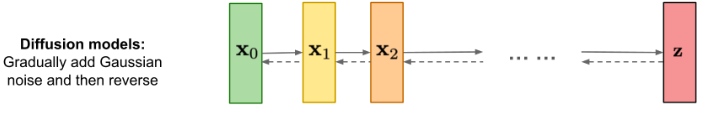A high level view of Diffusion Models is shown in Figure gen1. A fully trained model generates images by taking a Latent Variable consisting of random noise data ${\bf z}$ to the right of the figure, and gradually 'de-noises' it in multiple stages as shown by the dotted arrows moving from right to left (typically 1000 stages in large networks such as DALL-E-2). This is done by feeding the output of stage $t$ to stage $t-1$, until we get to the final stage resulting in the image ${\bf x_0}$. This is illustrated in Figure gen2, with the middle row illustrating the de-noising process starting from random data in stage $T$, followed by partially re-constructed image at stage ${T\over 2}$ and the final image at stage $0$.

Diffusion Models are trained by running the process just described in the opposite direction, i.e., from left to right in Figure gen1, as shown by the solid arrows. An image ${\bf x_0}$ from the training dataset is fed into stage 1 resulting in image ${\bf x_1}$. This stage as well as the following ones add small amounts of noise to the image, so it becomes progressively more and more blurry, until all that is left at state $T$ is pure noise (this is illustrated in the top row of Figure gen2. Since we know precisely how much noise is being added in each stage, the DDPM algorithm works by training a DLN to predict the added noise level given the noisy image data as the input into the model. This optimization problem is posed as that of minimizing a convex regression loss, which is a much simpler problem to solve as compared to the minmax approach in GANs.

In :
#gen2
nb_setup.images_hconcat(["DL_images/gen2.png"], width=1000)

Out: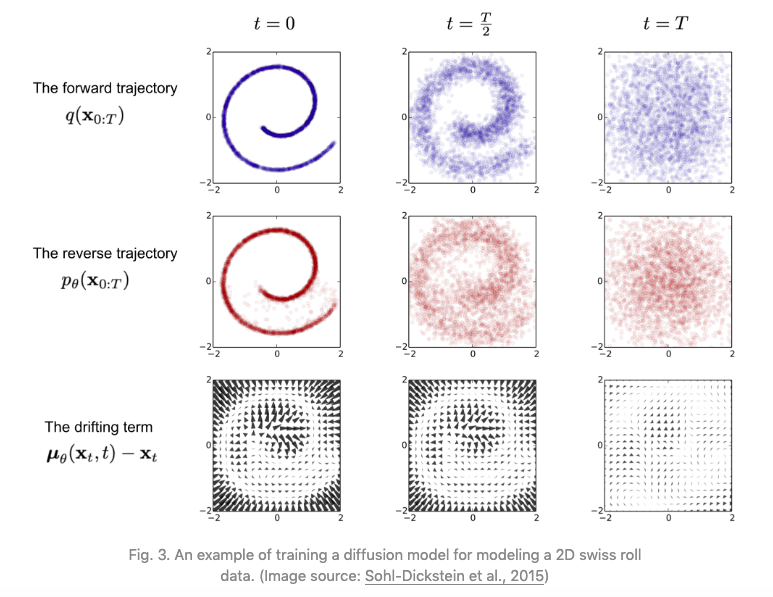### Latent Variables and the ELBO Bound¶

In :
#gen11
nb_setup.images_hconcat(["DL_images/gen11.png"], width=1000)

Out: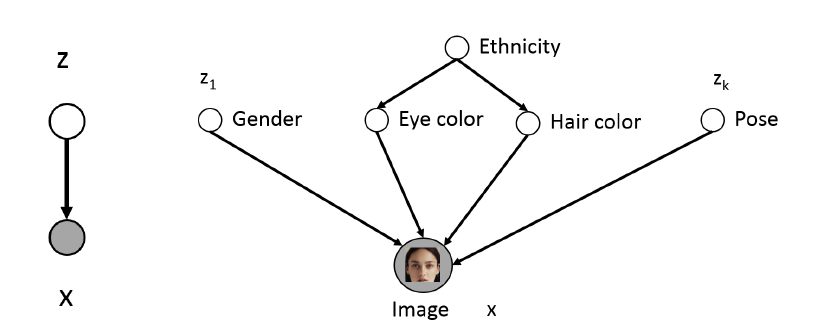Given a set of image samples $(X_1,...,X_N)$ we would like to estimate their Probability Density functions $q(X)$, so that we can sample from it and thereby generate new images. However, in general $q(X)$ is a very complex function, and a simpler problem is to define a Latent Variable $Z$ that encodes semantically useful information about $X$ and then estimate $q(X|Z)$ with the hope that this will be a simpler function to estimate. The idea behind Latent Variables is illustrated in Figure gen11: The LHS of the figure shows how new images can be generated in a two step process: First sample a Latent Variable $Z$ assuming that we know its distribution $q(Z)$ and then sample from $q(X|Z)$ to generate a new image. The RHS of the figure gives some intuition behind the concept of Latent Variables, it shows how the contents of an image of a human face are controlled by variables such as gender, hair color etc, and if these variables are specified, then the face generation problem becomes simpler.

In :
#gen12
nb_setup.images_hconcat(["DL_images/gen12.png"], width=1000)

Out: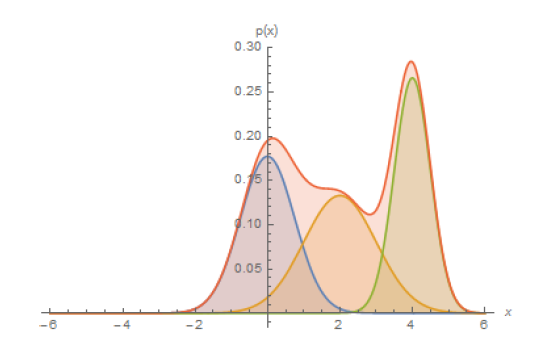For another perspective into the problem, consider Figure gen12 which shows the data distribution $q(X)$ which in general can a very complicated function (as a stand-in for an image distribution). However note that by using the Law of Total Probabilities, $q(X)$ can also written as $$q(X) = \int q(X,Z) dZ = \int q(X|Z) q(Z) dZ$$ If $q(X|Z)$ is a simpler function that can be approximated by a Gaussian $p_\theta(X|Z) = p_\theta(\mu_\theta(Z),\sigma_\theta(Z))$ then $$q(X) \approx \int p_\theta(\mu_\theta,\sigma_\theta) q(Z) dZ$$ Thus a complex $q(X)$ can be built by using a number of of these Gaussians super-imposed togther and weighted by the distribution $q(Z)$ as shown in Figure gen12. This is somewhat analogous to approximating a complex function by its Fourier Transform co-efficients which act as weights for simple sinusoidal functions.

We now show how to obtain the approximations $p_\theta(X|Z)$ by solving an optimization problem.

Evidence Lower Bound or ELBO is a useful technique from Bayesian Statistics, that has found use in Neural Network modeling in recent years. It works by converting the problem of estimating an unknown probability distribution into an optimization problem, which can then be solved using Neural Networks.

In :
#gen4
nb_setup.images_hconcat(["DL_images/gen4.png"], width=1000)

Out: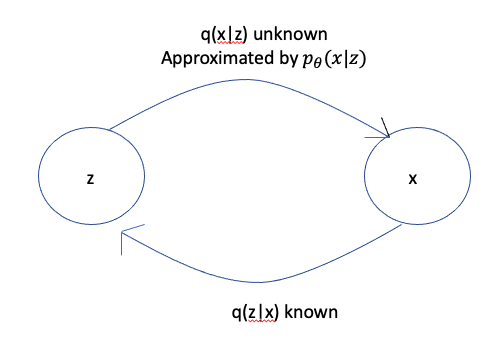Consider the following problem: Given two random variables $X$ and $Z$, the conditional probabilities $q(Z|X)$ and $q(Z)$ are known, and we are asked to invert the conditional probability and thus compute $q(X|Z)$. For example $X$ may be an image so that $Z$ is the Latent Variable representation of the image. In this case the problem can be formulated as: We know how to get the latent representation from the image, but we don't know how to convert a latent representation back into an image.

The most straightforward way of inverting the conditional probability is by means of Bayes Theorem, which states

$$q(X|Z) = {{q(Z|X)q(x)}\over{\sum_u q(Z|U)q(U)}}$$

However this formula is very difficult to compute because the sum in the denominator is often intractable. In order to solve this problem using Neural Networks, we have to turn this into an optimization problem. This is done by means of a technique called ELBO (Evidence Lower Bound) also known as VLB (Variational Lower Bound), which works as follows: Lets assume that we can approximate $q(X|Z)$ by another (parametrized) distribution $p_\theta(X|Z)$. In order to find the best $p_\theta(X|Z)$, we can try to minimize the "distance" between $p_\theta(X|Z)$ and $q(X|Z)$. A measure of distance between probability distributions is the Kullback-Leibler Divergence or KL Divergence. The KL Divergence between probability distributions $f(X)$ and $g(X)$ is defined as: $$D_{KL}(f(X), g(X)) = \sum f(X)\log{f(X)\over g(X)}$$ Substituting $f(X) = q(X|Z)$ and $g(X) = p_\theta(X|Z)$, and making use of the Law of Conditional Probabilities, it can be shown that $$D_{KL}(q(X|Z), p_\theta(X|Z)) = \log q(X) - \sum_Z q(Z|X)\log{ p_\theta(X,Z)\over q(Z|X)}$$ Hence in order to minimize $D_{KL}(q(X|Z), p_\theta(X|Z))$, we have to maximize $\sum_Z q(Z|X)\log{ p_\theta(X,Z)\over q(Z|X)}$, or minimize $\sum_Z q(Z|X)\log{q(Z|X)\over p_\theta(X,Z)}$. We will refer to the latter quantity as the ELBO or VLB, i.e., $$ELBO = \sum_Z q(Z|X)\log{q(Z|X)\over p_\theta(X,Z)} \tag 1$$

In :
#gen13
nb_setup.images_hconcat(["DL_images/gen13.png"], width=1000)

Out: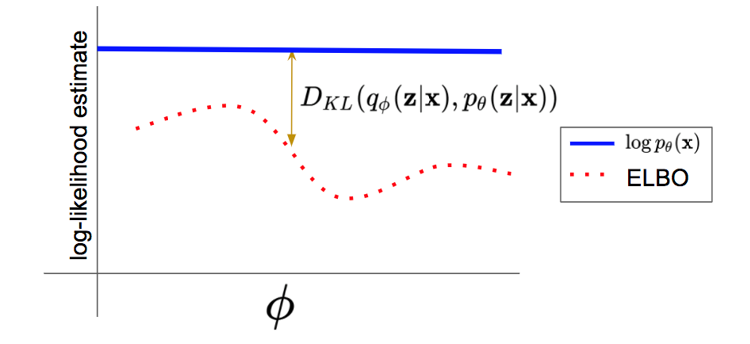Since by definition $D_{KL}(q(X|Z), p_\theta(X|Z)) \ge 0$, it follows that $$\log q(X) \ge ELBO$$ i.e., the ELBO serves as an approximation to the true distribution $q(X)$, with the difference betwen the two being equal to the KL Divergence $D_{KL}(q(X|Z), p_\theta(X|Z))$ as illustrated in Figure gen13.

Note: In Diffusion Approximations the distribution $q(Z|X)$ is known (as explained in the next section), however this is not the case in other Latent Variable models such as VAEs. In that case $q(Z|X)$ is also approximated by a parametrized distribution $q_\phi(Z|X)$, which is assumed to Gaussian as well. Thus building a VAE model requires a joint optimization of the parameters $(\theta,\phi)$.

Hence even though we don't have access to the true distribution $q(X)$, we can approximate it using the ELBO, with the parameters $\theta$ (or $\theta,\phi$) being chosen so as to minimize the difference between the two.

The ELBO serves as a convenient optimization measure that can be used to train a Neural Network. To make the problem more tractable, it is also assumed that the unknown distribution $p_\theta$ belongs to the family of Gaussian distributions $N(\mu_\theta,\sigma_\theta)$, which reduces the problem to that of estimating its mean $\mu_\theta$ and variance $\sigma_\theta$. These quantities can be estimated by using a DLN with parameters $\theta$, with the ELBO serving as a Loss Function to optimize the network. This optimization procedure underlies Diffusion Models.

### Forward Diffusion Process¶

In :
#gen3
nb_setup.images_hconcat(["DL_images/gen3.png"], width=1000)

Out: